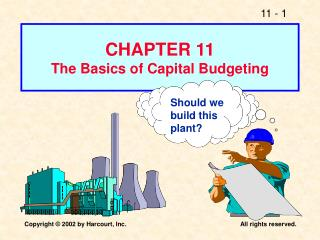DownloadDownload PresentationShould we build this plant?

# Should we build this plant?

Télécharger la présentation## Should we build this plant?

- - - - - - - - - - - - - - - - - - - - - - - - - - - E N D - - - - - - - - - - - - - - - - - - - - - - - - - - -
##### Presentation Transcript

1. CHAPTER 11The Basics of Capital Budgeting Should we build this plant?

2. What is capital budgeting? • Analysis of potential additions to fixed assets. • Long-term decisions; involve large expenditures. • Very important to firm’s future.

3. Steps 1. Estimate CFs (inflows & outflows). 2. Assess riskiness of CFs. 3. Determine k = WACC. 4. Find NPV and/or IRR. 5. Accept if NPV > 0 and/or IRR > WACC.

4. What is the difference between independent and mutually exclusive projects? Projects are: independent, if the cash flows of one are unaffected by the acceptance of the other. mutually exclusive, if the cash flows of one can be adversely impacted by the acceptance of the other.

5. An Example of Mutually Exclusive Projects BRIDGE vs. BOAT to get products across a river.

6. Normal Cash Flow Project: Normal Cash Flow Project Cost (negative CF) followed by a series of positive cash inflows. One change of signs. Nonnormal Cash Flow Project Two or more changes of signs. Most common: Cost (negative CF), then string of positive CFs, then cost to close project. Nuclear power plant, strip mine.

7. Inflow (+) or Outflow (-) in Year 0 1 2 3 4 5 N NN - + + + + + N - + + + + - NN - - - + + + N + + + - - - N - + + - + - NN

8. NPV: Sum of the PVs of inflows and outflows.

9. What is Project L’s NPV? Project L: 0 1 2 3 10% -100.00 10 60 80 9.09 49.59 60.11 18.79 = NPVL

10. Calculator Solution Enter in CFLO for L: -100 10 60 80 10 CF0 CF1 CF2 CF3 I NPV = 18.78 = NPVL

11. What is Project S’s NPV? Project S: 0 1 2 3 10% -100.00 70 50 20 63.64 41.32 15.03 19.99 = NPVS

12. Calculator Solution Enter in CFLO for S: -100 70 50 20 10 CF0 CF1 CF2 CF3 I NPV = 19.98 = NPVS

13. Rationale for the NPV Method NPV = PV inflows – Cost = Net gain in wealth. Accept project if NPV > 0. Choose between mutually exclusive projects on basis of higher NPV. Adds most value.

14. Using NPV method, which project(s) should be accepted? • If Projects S and L are mutually exclusive, accept S because NPVs > NPVL . • If S & L are independent, accept both; NPV > 0.

15. Internal Rate of Return: IRR 0 1 2 3 CF0 CF1 CF2 CF3 Cost Inflows IRR is the discount rate that forces PV inflows = cost. This is the same as forcing NPV = 0.

16. NPV: Enter k, solve for NPV. IRR: Enter NPV = 0, solve for IRR.

17. Enter CFs in CFLO, then press IRR: IRRL = 18.13%. What’s Project L’s IRR? 0 1 2 3 IRR = ? -100.00 10 60 80 PV1 PV2 PV3 0 = NPV

18. Finding IRR Without a Financial Calculator Find the NPV first. If the NPV is positive, then try an IRR > WACC. Project L, Try 20%: 100 =(10*1/1.2)+(60*1/(1.2)2) +(80*1/(1.2)3) 100=96.30 Need higher PV, so lower IRR Answer 10%<IRR<20%, accept (WACC = 10%)

19. Enter CFs in CFLO, then press IRR: IRRS = 23.56%. What’s Project S’s IRR? 0 1 2 3 IRR = ? -100.00 70 50 20 PV1 PV2 PV3 0 = NPV

20. Finding IRR Without a Financial Calculator Find the NPV first. If the NPV is positive, then try an IRR > WACC. Project S, Try 12%: 100 =(70*1/1.12)+(50*1/(1.12)2) +(20*1/(1.12)3) 100=116.60 Need lower PV, so higher IRR Answer IRR>12%, accept (WACC = 10%)

21. Q. How is a project’s IRR related to a bond’s YTM? A. They are the same thing. A bond’s YTM is the IRR if you invest in the bond. 0 1 2 10 IRR = ? ... -1134.2 90 90 1090 IRR = 7.08% (use TVM or CFLO).

22. Rationale for the IRR Method If IRR > WACC, then the project’s rate of return is greater than its cost--some return is left over to boost stockholders’ returns. Example: WACC = 10%, IRR = 15%. Profitable.

23. IRR Acceptance Criteria • If IRR > k, accept project. • If IRR < k, reject project.

24. Decisions on Projects S and L per IRR • If S and L are independent, accept both. IRRs > k = 10%. • If S and L are mutually exclusive, accept S because IRRS > IRRL .

25. Prefer NPV to IRR • Can have multiple IRR’s if you have non-normal cash flows. • Can get different results depending on the WACC used when ranking projects using NPV and IRR. • Reinvestment rate assumption makes more sense with NPV than with IRR.

26. Reinvestment Rate Assumptions • NPV assumes reinvest at k (opportunity cost of capital). • IRR assumes reinvest at IRR. • Reinvest at opportunity cost, k, is more realistic, so NPV method is best. NPV should be used to choose between mutually exclusive projects.